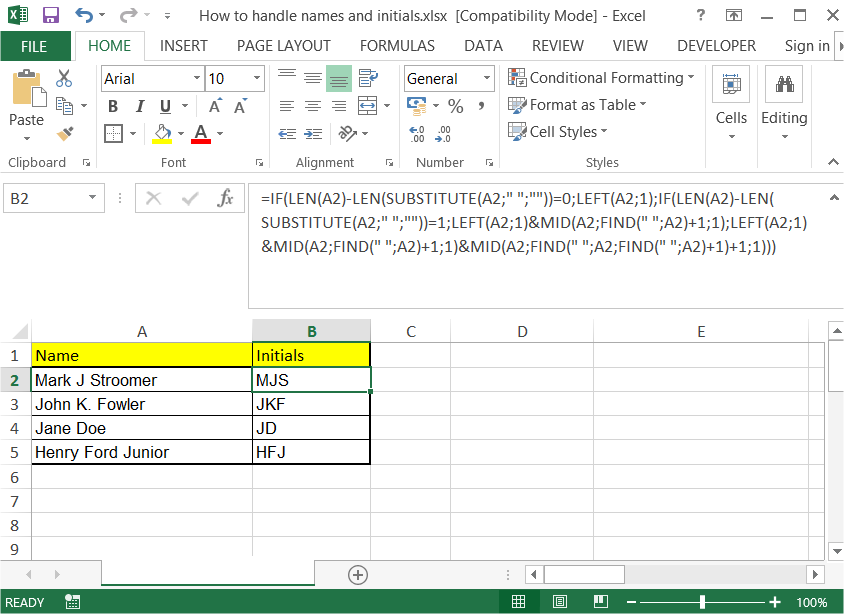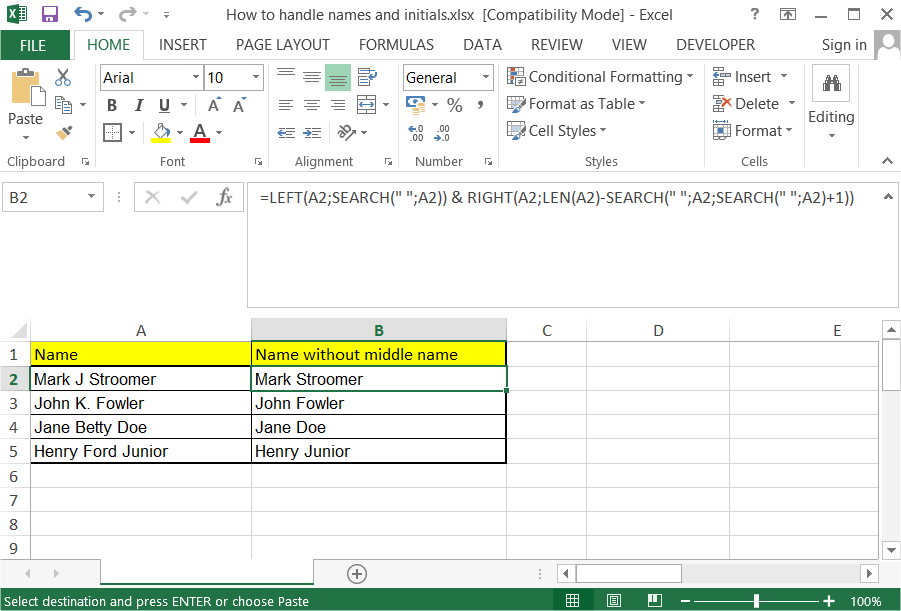### How to Handle Names and Initials in Excel

There are many times when initials made me crazy. In this article you can teach yourself how to handle initials with Excel. A few tricks for you here.

A few tricks for you how to handle initials within Excel. All of them combined in this article.

### Getting initials from a name

Let’s start from the easiest one. When only two-lettered initials needed just use:

=LEFT(\$A1)&MID(\$A1,FIND(” “,\$A1)+1,1)

This formula will extract you initials of first name and surname. Two letters only.### Getting initials from a first name, middle name and surname

More advanced one. Use this one when always first name, middle name and surname provided. Below one will help you:

=LEFT(\$A1)&MID(\$A1,FIND(” “,\$A1)+1,1)&MID(RIGHT(\$A1,LEN(\$A1)-FIND(” “,\$A1,1)),FIND(” “,RIGHT(,LEN(\$A1)-FIND(” “,\$A1,1)))+1,1)

This formula give you three initial letters. It will not work when no middle name provided.### Getting initials from full name

This is a complex formula which you can use to extract initials no matter what exactly you have in your data.

=IF(LEN(\$A1)-LEN(SUBSTITUTE(\$A1,” “,””))=0,LEFT(\$A1,1),IF(LEN(\$A1)-LEN(SUBSTITUTE(\$A1,” “,””))=1,LEFT(\$A1,1)&MID(\$A1,FIND(” “,\$A1)+1,1),LEFT(\$A1,1)&MID(\$A1,FIND(” “,\$A1)+1,1)&MID(\$A1,FIND(” “,\$A1,FIND(” “,\$A1)+1)+1,1)))

This formula is complicated but works every time.### Getting first name and surname (without middle name)

Use below formula when you don’t care about middle names.

=LEFT(\$A1,SEARCH(” “,\$A1))&RIGHT(\$A1,LEN(\$A1)-SEARCH(” “,\$A1,SEARCH(” “,\$A1)+1))

This formula simplifies your data and cuts middle names off.### Changing order of first name, middle name and surname

Thanks to this formula you can change the order. In my example you will get: surname coma space first name initial of middle name

=RIGHT(\$A1,LEN(G2)-FIND(“.”,\$A1)-1)&”, “&LEFT(\$A1,FIND(“.”,\$A1))I hope this article makes your life easier. Thanks to it you will handle names and initials easier.

## Template

```Further reading: